# Lecture 17 Flow Analysis flow analysis in prolog

• Slides: 68Lecture 17 Flow Analysis flow analysis in prolog; applications of flow analysis Ras Bodik Shaon Barman Thibaud Hottelier Hack Your Language! CS 164: Introduction to Programming Languages and Compilers, Spring 2012 UC Berkeley 1Today Static program analysis what is it and why do it Points-to analysis static analysis for understanding how pointer values flow Andersen’s algorithm via deduction Andersen’s algorithm in Prolog just four lines Andersen’s algorithm via CYK parsing (optional) CFL-reachability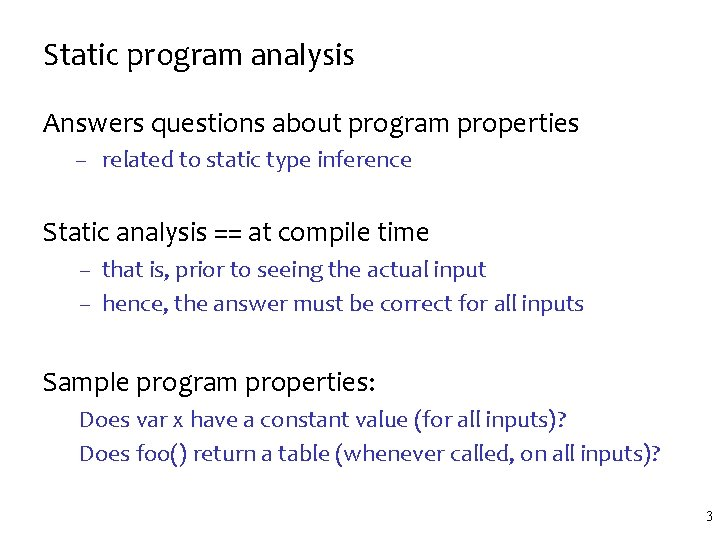Static program analysis Answers questions about program properties – related to static type inference Static analysis == at compile time – that is, prior to seeing the actual input – hence, the answer must be correct for all inputs Sample program properties: Does var x have a constant value (for all inputs)? Does foo() return a table (whenever called, on all inputs)? 3Motivation for static program analysis (1) Optimize the program. Ex: replace x[i] with x if we know that i is always 1. Constant propagation i=2 … i = i+2 … if (…) { …} … x[i] = x[i-1] 4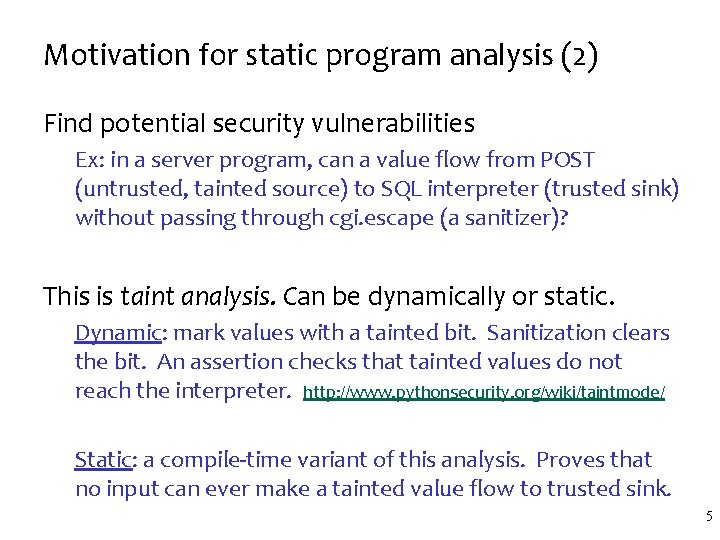Motivation for static program analysis (2) Find potential security vulnerabilities Ex: in a server program, can a value flow from POST (untrusted, tainted source) to SQL interpreter (trusted sink) without passing through cgi. escape (a sanitizer)? This is taint analysis. Can be dynamically or static. Dynamic: mark values with a tainted bit. Sanitization clears the bit. An assertion checks that tainted values do not reach the interpreter. http: //www. pythonsecurity. org/wiki/taintmode/ Static: a compile-time variant of this analysis. Proves that no input can ever make a tainted value flow to trusted sink. 5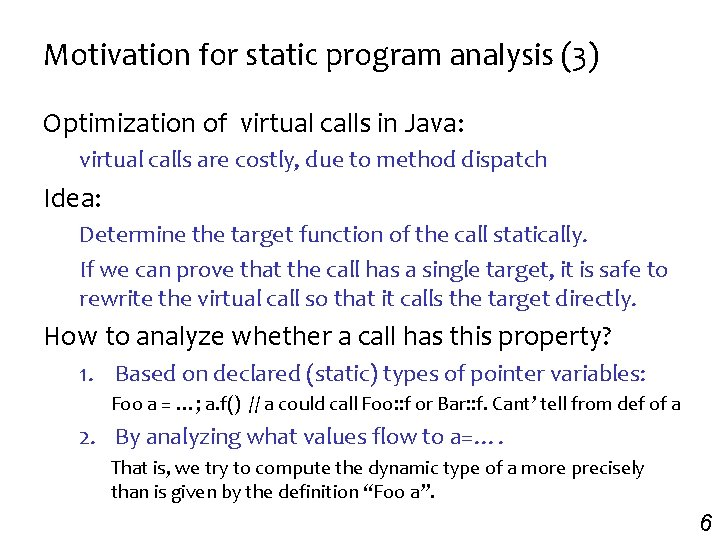Motivation for static program analysis (3) Optimization of virtual calls in Java: virtual calls are costly, due to method dispatch Idea: Determine the target function of the call statically. If we can prove that the call has a single target, it is safe to rewrite the virtual call so that it calls the target directly. How to analyze whether a call has this property? 1. Based on declared (static) types of pointer variables: Foo a = …; a. f() // a could call Foo: : f or Bar: : f. Cant’ tell from def of a 2. By analyzing what values flow to a=…. That is, we try to compute the dynamic type of a more precisely than is given by the definition “Foo a”. 6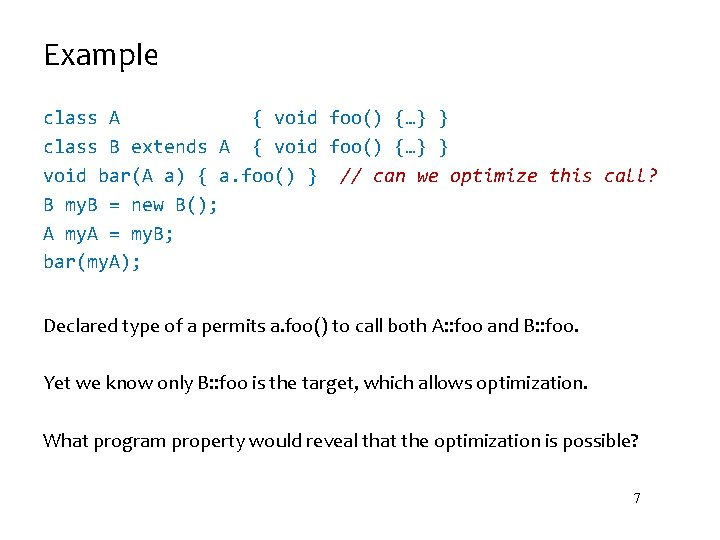Example class A { void foo() {…} } class B extends A { void foo() {…} } void bar(A a) { a. foo() } // can we optimize this call? B my. B = new B(); A my. A = my. B; bar(my. A); Declared type of a permits a. foo() to call both A: : foo and B: : foo. Yet we know only B: : foo is the target, which allows optimization. What program property would reveal that the optimization is possible? 7Client 2: Verification of casts In Java, casts are checked at run time – type system not expressive enough to check them statically – although Java generics help somewhat The anatomy of a cast check: (Foo) e translates to – if ( dynamic_type_of(e) not compatible with Foo ) throw Class. Cast Exception – t 1 compatible with t 2: t 1 = t 2 or t 1 subclass of t 2 Goal: prove that no exception will happen at runtime – Why do this? The exception prevents any security holes, no? – Such static verification useful to catch bugs (Mars Rover). 8Example class Simple. Container { Object a; void put (Object o) { a=o; } Object get() { return a; } } Simple. Container c 1 = new Simple. Container(); Simple. Container c 2 = new Simple. Container(); c 1. put(new Foo()); c 2. put(“Hello”); Foo my. Foo = (Foo) c 1. get(); // verify that cast does not fail Note: analysis must distinguish containers c 1 and c 2. – otherwise c 1 will appear to contain string objects What property will lead to desired verification? 9Motivation for static program analysis (4) Compile 164 into efficient code If p always refers to tables that contains fields f 1 and f 2, we can represent the table as a struct and compile p[“f 2”] into an (efficient) instruction “load from address in p + 4 bytes”. The analysis Determine at compile time what fields the object may ever contain at run time. A conservative rule (conservative=sufficient but not necessary): Compute, at compile time: • the set of fields are added to the table using stmt e. ID=e • the table’s fields must not be written or read through operator e[e] (only through e. ID) 10Discussion Why is e[e] dangerous? Consider: – p[read_input_string()]=… creates a field whose name is unknown statically 11Example (Java. Script) var p = new Foo; // line 1 var r = p. field; var s = {}; s[r. f] = p; var t = s[input()]; t. g = … Consider the Foo objects created in line 1: Can we determine at compile time what fields these objects will contain during their lifetime (for any input)? If these objects are not accessed via e[e], then we can compute (a superset of) these fields. Can we tell if this program access Foo’s via e[e]? 12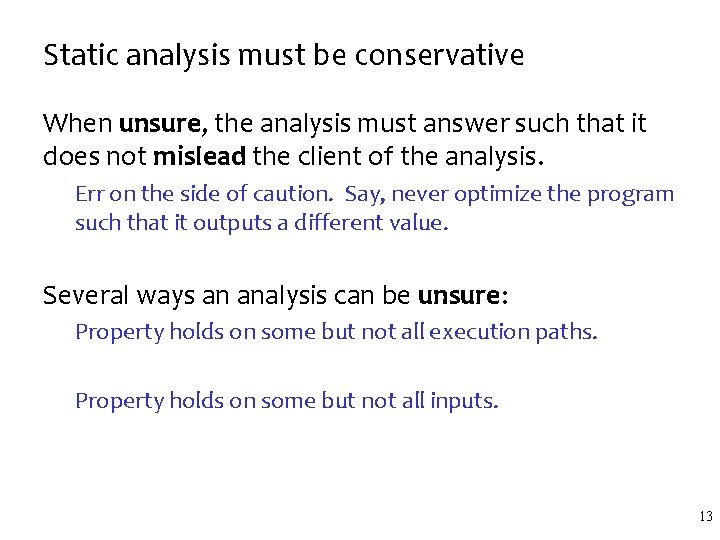Static analysis must be conservative When unsure, the analysis must answer such that it does not mislead the client of the analysis. Err on the side of caution. Say, never optimize the program such that it outputs a different value. Several ways an analysis can be unsure: Property holds on some but not all execution paths. Property holds on some but not all inputs. 13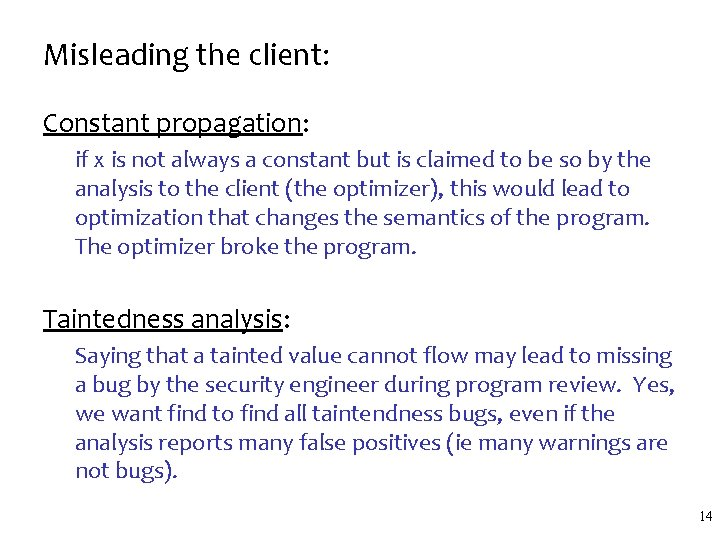Misleading the client: Constant propagation: if x is not always a constant but is claimed to be so by the analysis to the client (the optimizer), this would lead to optimization that changes the semantics of the program. The optimizer broke the program. Taintedness analysis: Saying that a tainted value cannot flow may lead to missing a bug by the security engineer during program review. Yes, we want find to find all taintendness bugs, even if the analysis reports many false positives (ie many warnings are not bugs). 14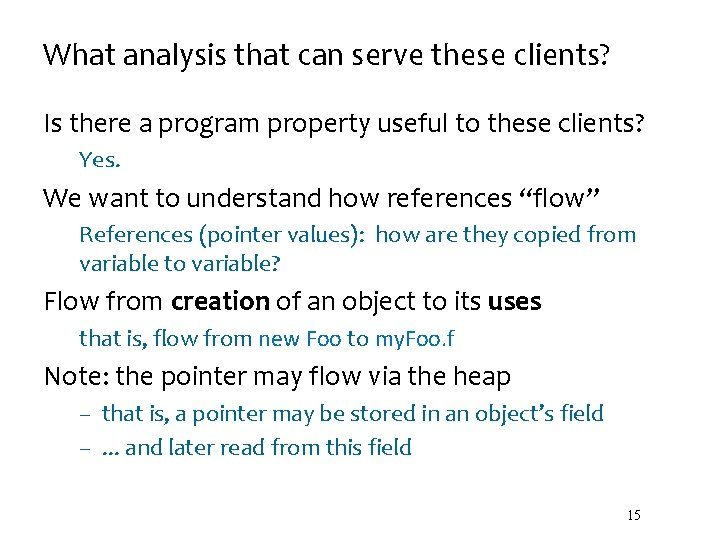What analysis that can serve these clients? Is there a program property useful to these clients? Yes. We want to understand how references “flow” References (pointer values): how are they copied from variable to variable? Flow from creation of an object to its uses that is, flow from new Foo to my. Foo. f Note: the pointer may flow via the heap – that is, a pointer may be stored in an object’s field –. . . and later read from this field 15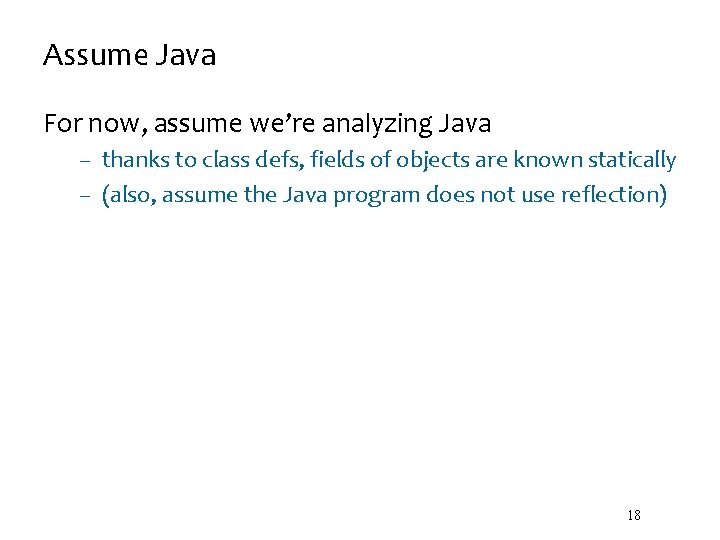Assume Java For now, assume we’re analyzing Java – thanks to class defs, fields of objects are known statically – (also, assume the Java program does not use reflection) 18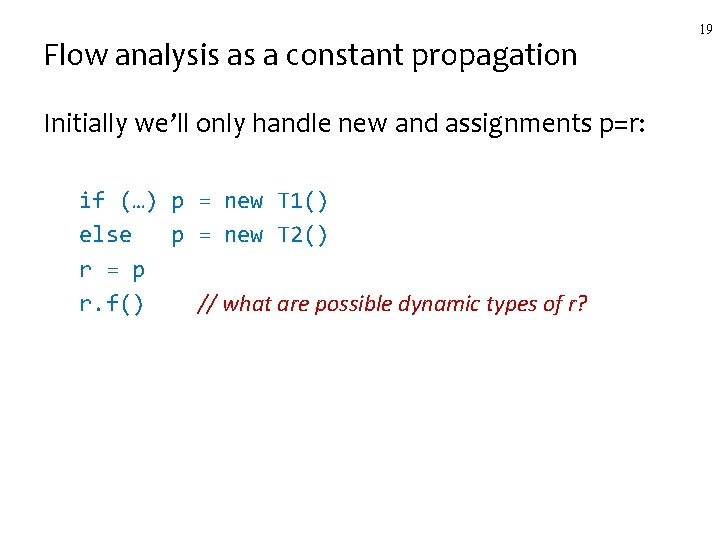Flow analysis as a constant propagation Initially we’ll only handle new and assignments p=r: if (…) p = new T 1() else p = new T 2() r = p r. f() // what are possible dynamic types of r? 19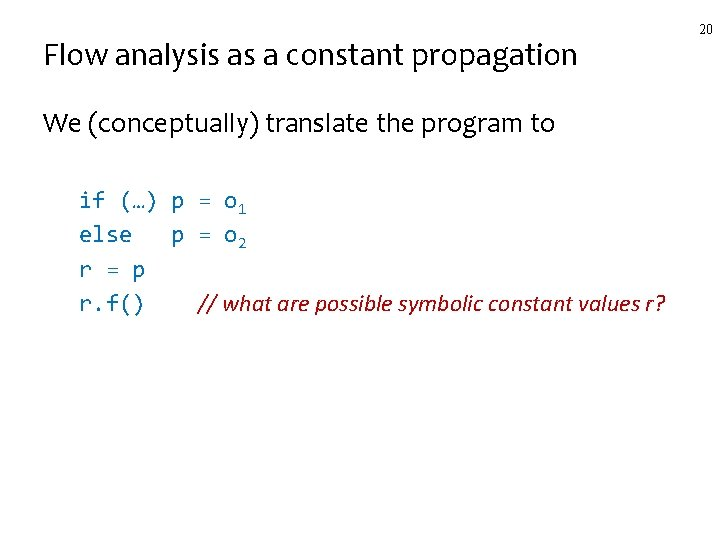Flow analysis as a constant propagation We (conceptually) translate the program to if (…) p = o 1 else p = o 2 r = p r. f() // what are possible symbolic constant values r? 20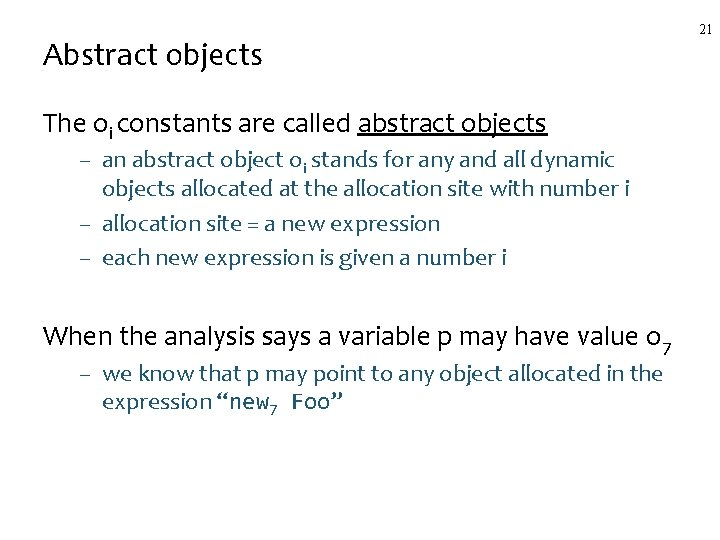Abstract objects The oi constants are called abstract objects – an abstract object oi stands for any and all dynamic objects allocated at the allocation site with number i – allocation site = a new expression – each new expression is given a number i When the analysis says a variable p may have value o 7 – we know that p may point to any object allocated in the expression “new 7 Foo” 21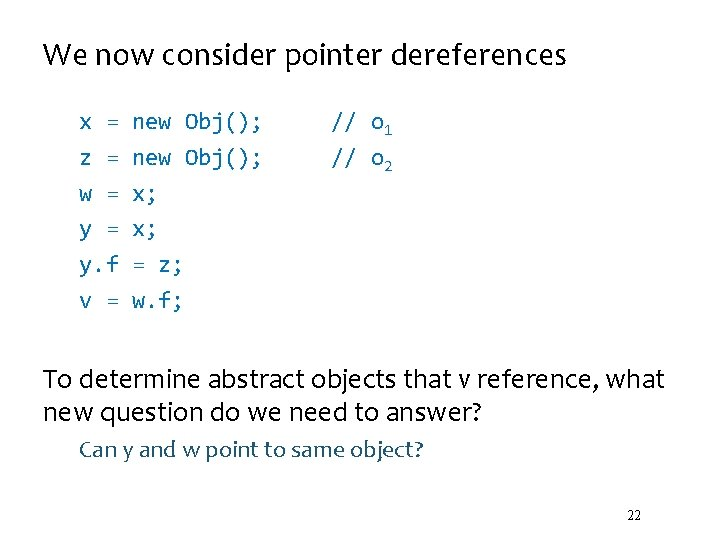We now consider pointer dereferences x = z = w = y. f v = new Obj(); x; x; = z; w. f; // o 1 // o 2 To determine abstract objects that v reference, what new question do we need to answer? Can y and w point to same object? 22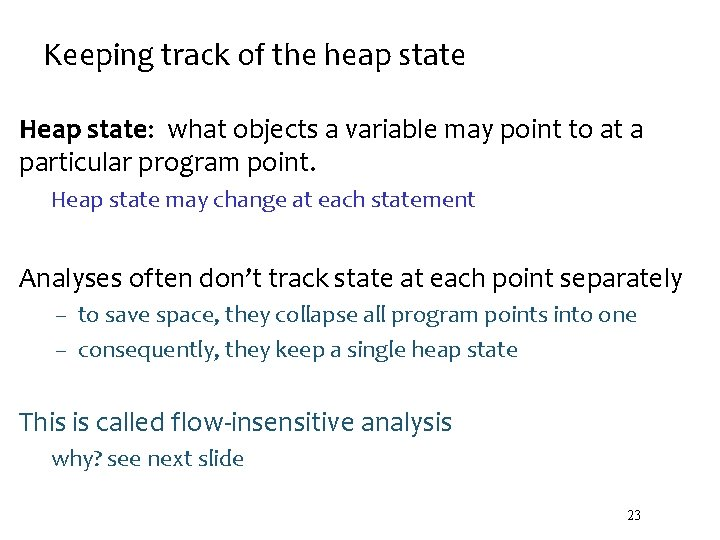Keeping track of the heap state Heap state: what objects a variable may point to at a particular program point. Heap state may change at each statement Analyses often don’t track state at each point separately – to save space, they collapse all program points into one – consequently, they keep a single heap state This is called flow-insensitive analysis why? see next slide 23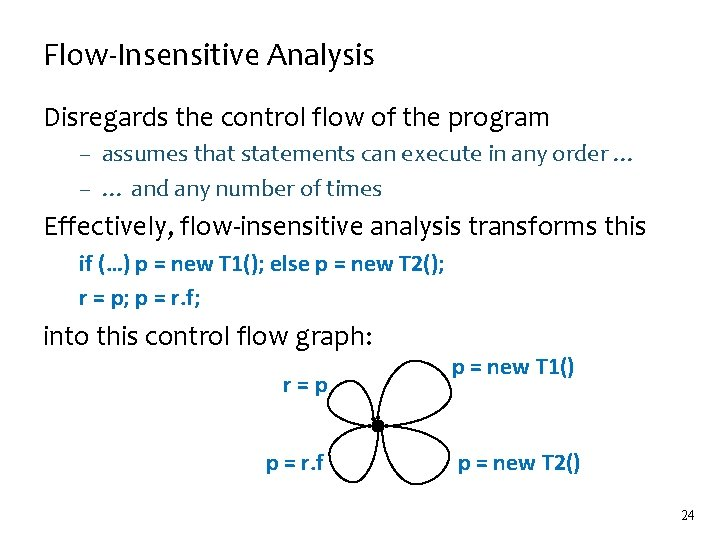Flow-Insensitive Analysis Disregards the control flow of the program – assumes that statements can execute in any order … – … and any number of times Effectively, flow-insensitive analysis transforms this if (…) p = new T 1(); else p = new T 2(); r = p; p = r. f; into this control flow graph: r=p p = r. f p = new T 1() p = new T 2() 24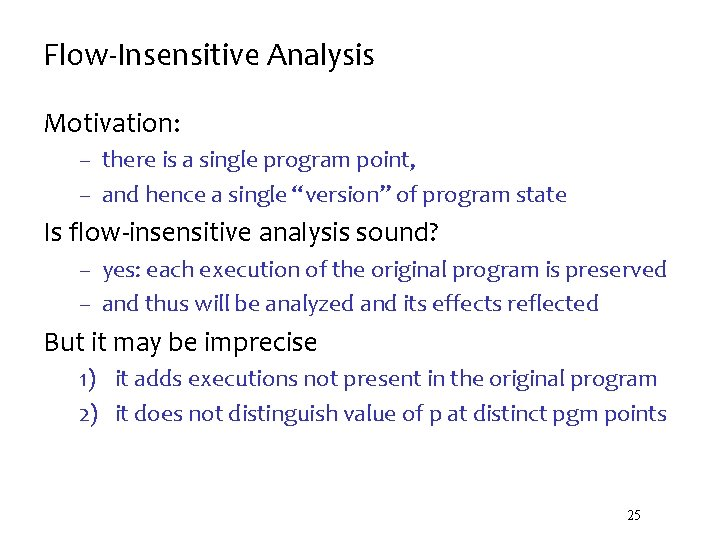Flow-Insensitive Analysis Motivation: – there is a single program point, – and hence a single “version” of program state Is flow-insensitive analysis sound? – yes: each execution of the original program is preserved – and thus will be analyzed and its effects reflected But it may be imprecise 1) it adds executions not present in the original program 2) it does not distinguish value of p at distinct pgm points 25Let’s develop the analysis! Canonical Stmts Java pointers give rise to complex expressions: – ex: p. f(). g. arr[i] = r. f. g(new Foo()). h Can we find a small set of canonical statements – ie, the core language understood by the analysis – we’ll desugar the rest of the program to these stmts We only need four canonical statements: p = new T() p=r p = r. f p. f = r new assign getfield putfield 26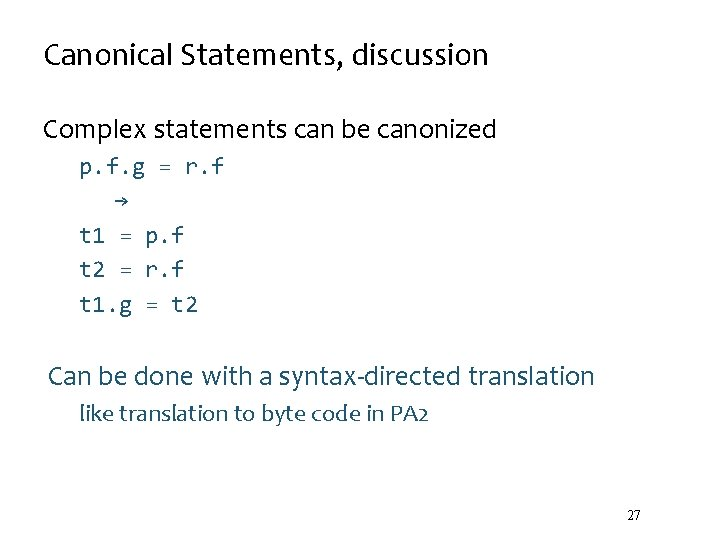Canonical Statements, discussion Complex statements can be canonized p. f. g = r. f → t 1 = p. f t 2 = r. f t 1. g = t 2 Can be done with a syntax-directed translation like translation to byte code in PA 2 27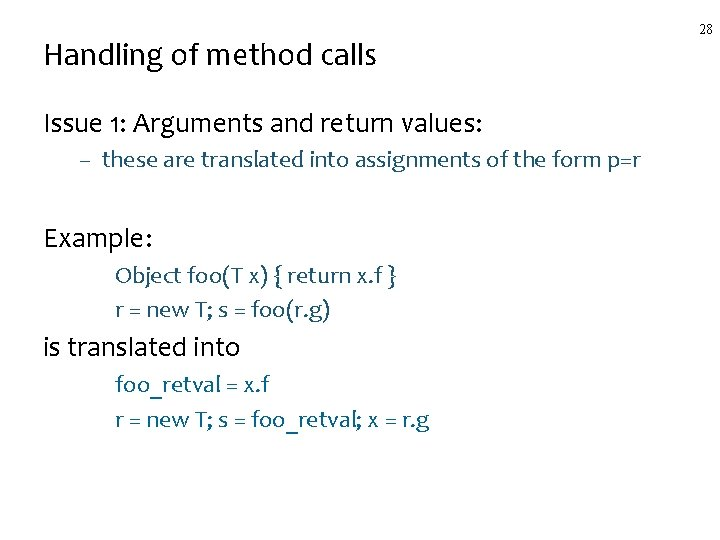Handling of method calls Issue 1: Arguments and return values: – these are translated into assignments of the form p=r Example: Object foo(T x) { return x. f } r = new T; s = foo(r. g) is translated into foo_retval = x. f r = new T; s = foo_retval; x = r. g 28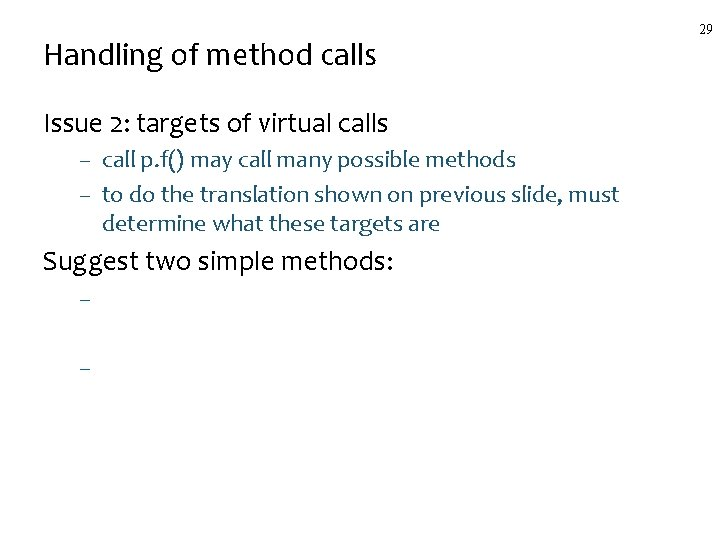Handling of method calls Issue 2: targets of virtual calls – call p. f() may call many possible methods – to do the translation shown on previous slide, must determine what these targets are Suggest two simple methods: – – 29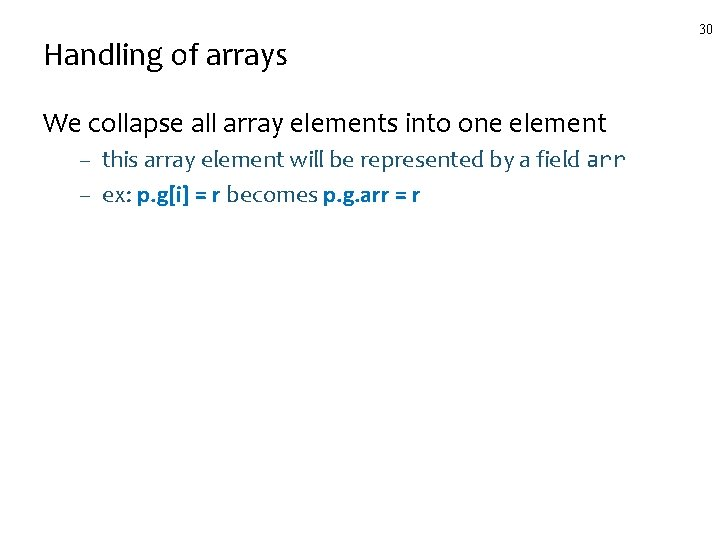Handling of arrays We collapse all array elements into one element – this array element will be represented by a field arr – ex: p. g[i] = r becomes p. g. arr = r 30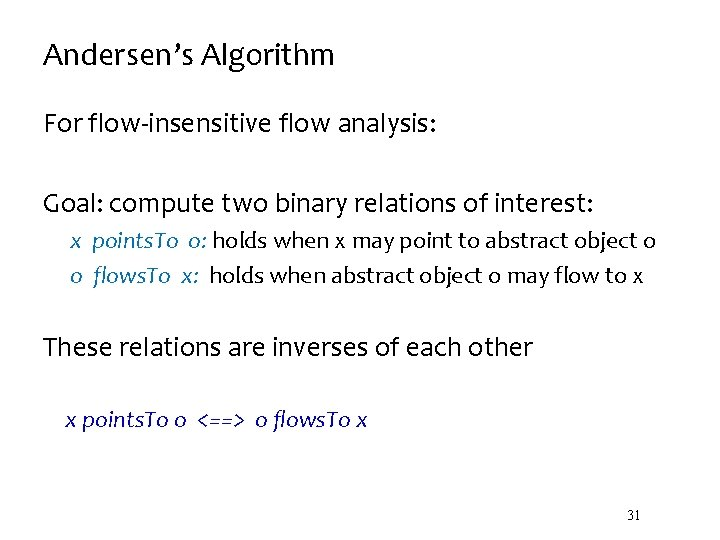Andersen’s Algorithm For flow-insensitive flow analysis: Goal: compute two binary relations of interest: x points. To o: holds when x may point to abstract object o o flows. To x: holds when abstract object o may flow to x These relations are inverses of each other x points. To o <==> o flows. To x 31These two relations support our clients These relations allows determining: 1. target methods of virtual calls 2. verification of casts 3. how Java. Script objects are used For 3) we need the flows. To relation For 1) and 2) we need the x points. To o relation 32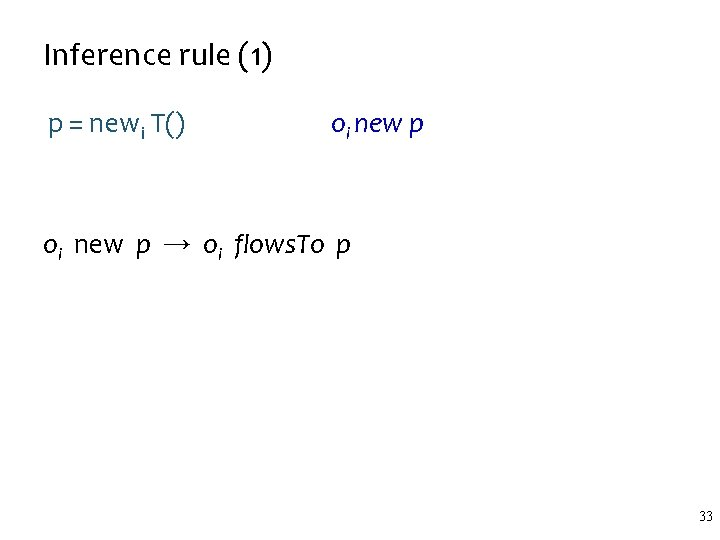Inference rule (1) p = newi T() oi new p → oi flows. To p 33Inference rule (2) p=r r assign p oi flows. To r �r assign p → oi flows. To p 34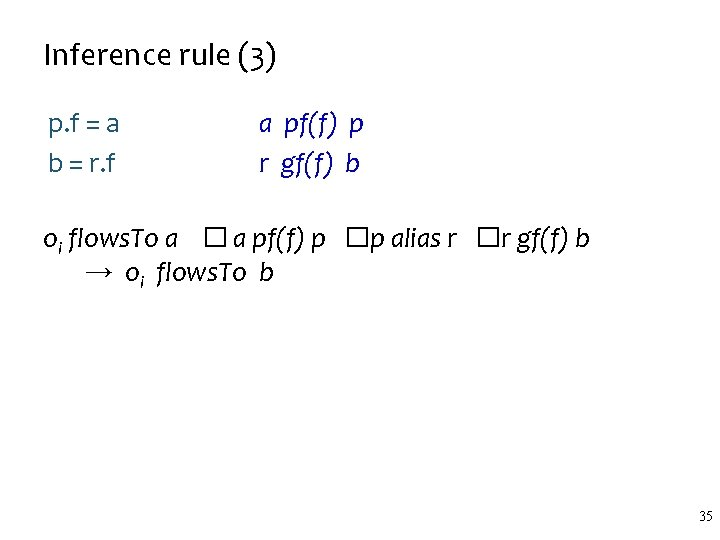Inference rule (3) p. f = a b = r. f a pf(f) p r gf(f) b oi flows. To a � a pf(f) p �p alias r �r gf(f) b → oi flows. To b 35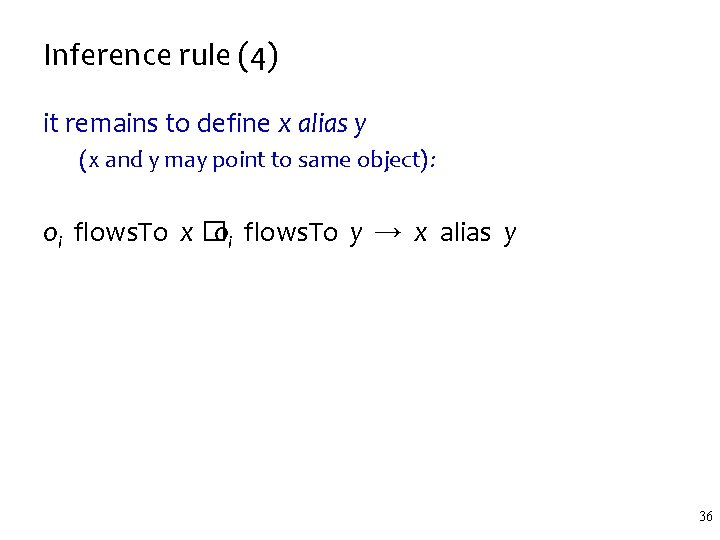Inference rule (4) it remains to define x alias y (x and y may point to same object): oi flows. To x � oi flows. To y → x alias y 36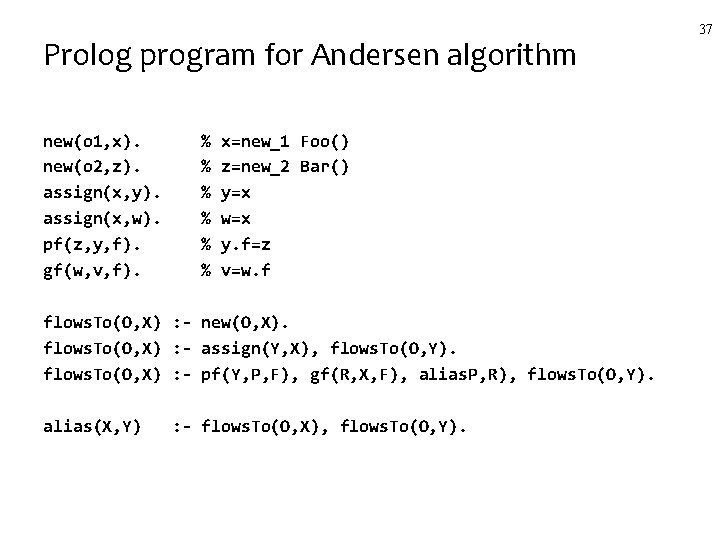Prolog program for Andersen algorithm new(o 1, x). new(o 2, z). assign(x, y). assign(x, w). pf(z, y, f). gf(w, v, f). % % % x=new_1 Foo() z=new_2 Bar() y=x w=x y. f=z v=w. f flows. To(O, X) : - new(O, X). flows. To(O, X) : - assign(Y, X), flows. To(O, Y). flows. To(O, X) : - pf(Y, P, F), gf(R, X, F), alias. P, R), flows. To(O, Y). alias(X, Y) : - flows. To(O, X), flows. To(O, Y). 37How to use the result of the analysis? When the analysis infers o flows. To y, what did we prove? – nothing useful, usually, since o flows. To y does not imply that there is a program input for which o will definitely flow to y. The useful result is when the analysis can’t infer o flows. To y – then we have proved that o cannot flow to y for any input – this is useful information! – it may lead to better optimization, verification, compilation Same arguments apply to alias, points. To relations – and other static analyses in general 38Inference Example (1) The program: x = new Foo(); // o 1 z = new Bar(); // o 2 w = x; y. f = z; v = w. f; 39Inference Example (2): The program is converted to six facts: o 1 new x x assign w z pf(f) y o 2 new z x assign y w gf(f) vInference Example (3), infering facts o 1 new x x assign w z pf(f) y o 2 new z x assign y w gf(f) v The inference: o 1 new x → o 1 flows. To x o 2 new z → o 2 flows. To z o 1 flows. To x �x assign w → o 1 flows. To w o 1 flows. To x �x assign y → o 1 flows. To y �o 1 flows. To w → y alias w o 2 flows. To z �z pf(f) y �y alias w �w gf(f) v → o 2 flows. To v. . . 41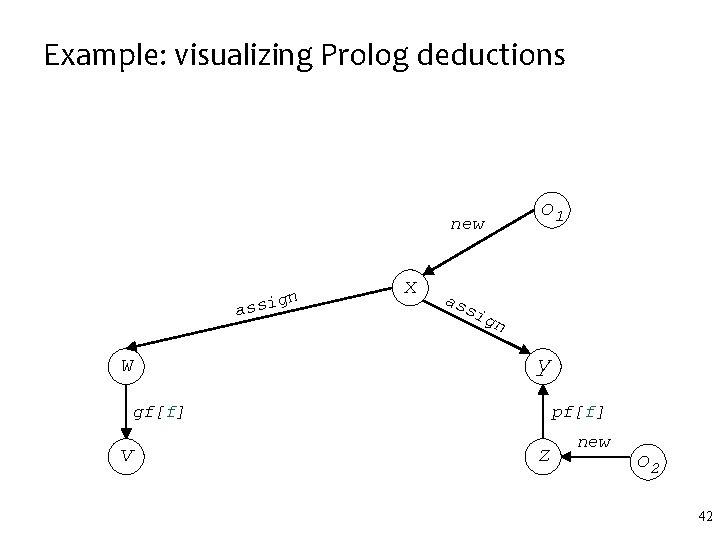Example: visualizing Prolog deductions new n ssig a w x o 1 as si gn y gf[f] v pf[f] z new o 2 42Example, deriving the relations new n ssig a w x o 1 as si gn y gf[f] v pf[f] z new o 2 43Example (4): Notes: – inference must continue until no new facts can be derived – only then we know we have performed sound analysis Conclusions from our example inference: – – – we have inferred o 2 flows. To v we have NOT inferred o 1 flows. To v hence we know v will point only to instances of Bar (assuming the example contains the whole program) thus casts (Bar) v will succeed similarly, calls v. f() are optimizable 44“Parsing the graph” Visualization of inferences on slides 41 and 42 parses the strings in the “graph of binary facts” using the CYK algorithm (Lecture 8) Details on this style of inference are in the rest of the slide, under CFL-reachability (optional material) 45Adaptation for Java. Script Need to handle more language constructs: – – property read e 1[e 2] property write e 1[e 2] = e 3 assume that e 2 can return any value, and the analysis does not analyze the value Extensions to the algorithm: - analysis must determine whether an object might appear as e 1 in e 1[e 2] = e 3 - if yes, we must conservatively assume that we don’t know objects fields - more similar rules are needed … 46Summary Determine run-time properties of programs statically – example property: “is variable x a constant? ” Statically: without running the program – it means that we don’t know the inputs – and thus must consider all possible program executions We want sound analysis: err on the side of caution. – allowed to say x is not a constant when it is – not allowed to say x is a constant when it is not Static analysis has many clients – optimization, verification, compilation 47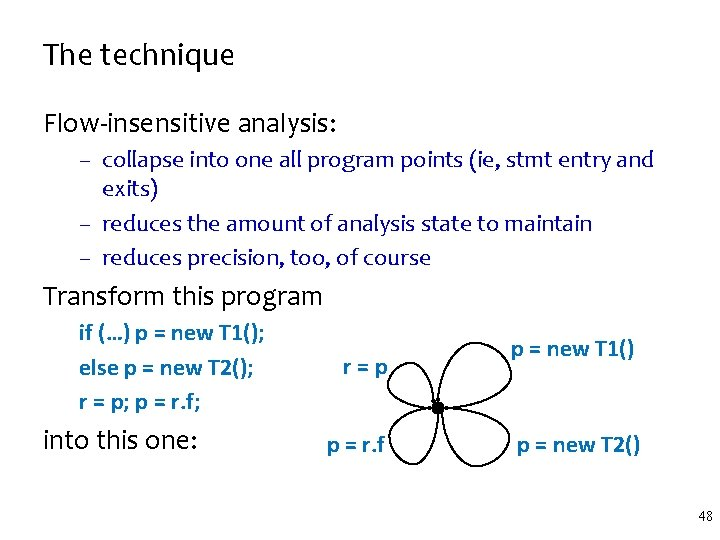The technique Flow-insensitive analysis: – collapse into one all program points (ie, stmt entry and exits) – reduces the amount of analysis state to maintain – reduces precision, too, of course Transform this program if (…) p = new T 1(); else p = new T 2(); r = p; p = r. f; into this one: r=p p = r. f p = new T 1() p = new T 2() 48Andersen’s algorithm • Deduces the flows. To relation from program statements – statements are facts – analysis is a set of inference rules – flows. To relation is a set of facts inferred with analysis rules • Statement facts: we’ll write them as x predicate. Name y – – p = newi T() p=r p = r. f p. f = r oi new p r assign p r gf(f) p r pf(f) p 49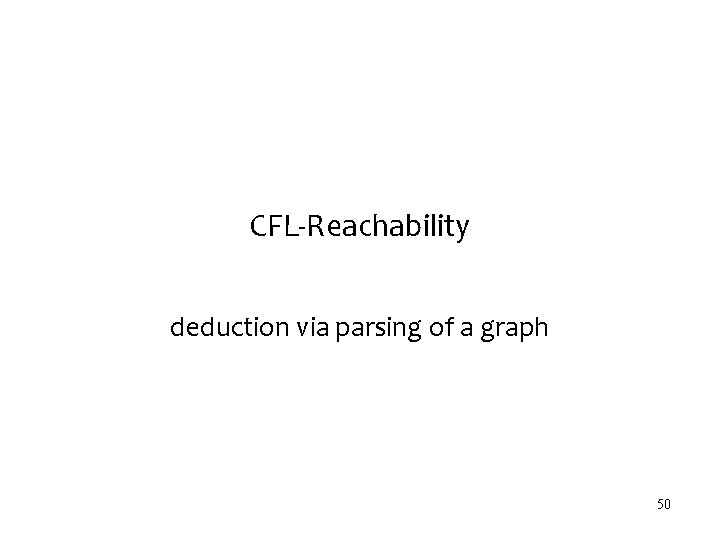CFL-Reachability deduction via parsing of a graph 50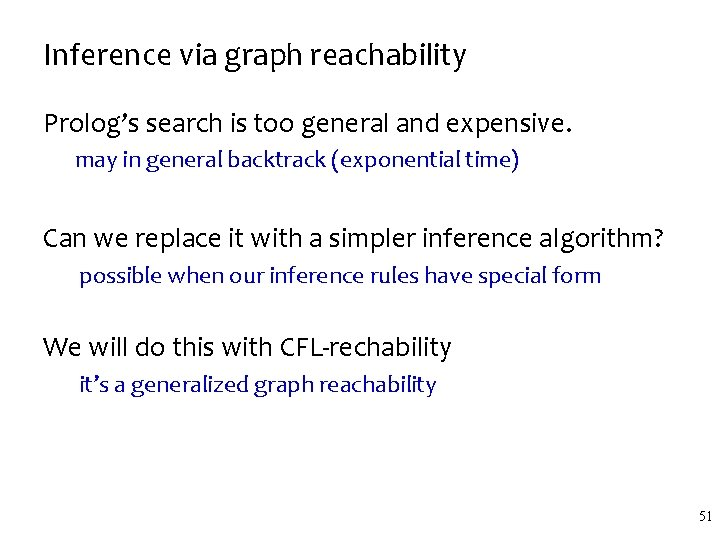Inference via graph reachability Prolog’s search is too general and expensive. may in general backtrack (exponential time) Can we replace it with a simpler inference algorithm? possible when our inference rules have special form We will do this with CFL-rechability it’s a generalized graph reachability 51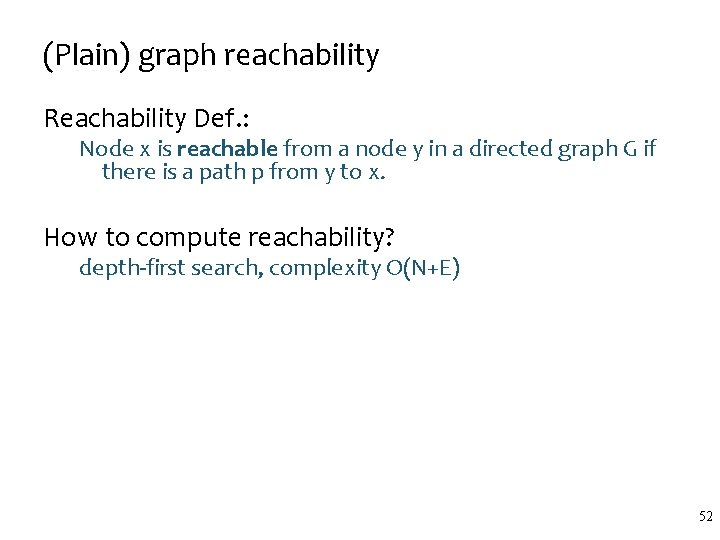(Plain) graph reachability Reachability Def. : Node x is reachable from a node y in a directed graph G if there is a path p from y to x. How to compute reachability? depth-first search, complexity O(N+E) 52Context-Free-Language-Reachability CFL-Reachability Def. : Node x is L-reachable from a node y in a directed labeled graph G if – there is a path p from y to x, and – path p is labeled with a string from a context free language L. e ) e s [ ( The context-free language L: ] e [ e t ] ] matched → matched | ( matched ) | [ matched ] | e | Is t reachable from s according to the language L? 53Computing CFL-reachability Given – a labeled directed graph P and – a grammar G with a start nonterminal S, we want to compute whether x is S-reachable from y – for all pairs of nodes x, y – or for a particular x and all y – or for a given pair of nodes x, y We can compute CFL-reachability with CYK parser – x is S-reachable from y if CYK adds an S-labeled edge from y to x – O(N 3) time 54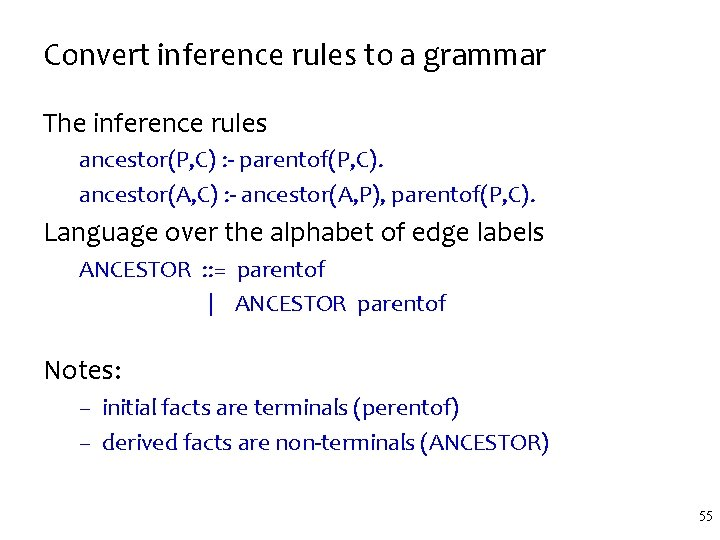Convert inference rules to a grammar The inference rules ancestor(P, C) : - parentof(P, C). ancestor(A, C) : - ancestor(A, P), parentof(P, C). Language over the alphabet of edge labels ANCESTOR : : = parentof | ANCESTOR parentof Notes: – initial facts are terminals (perentof) – derived facts are non-terminals (ANCESTOR) 55So, which rules can be converted to CFL-reachability? ANCESTOR : : = parentof | ANCESTOR parentof Is “son” ANCESTOR-reachable from “grandma”? grandma mom me son parentof ANCESTOR 56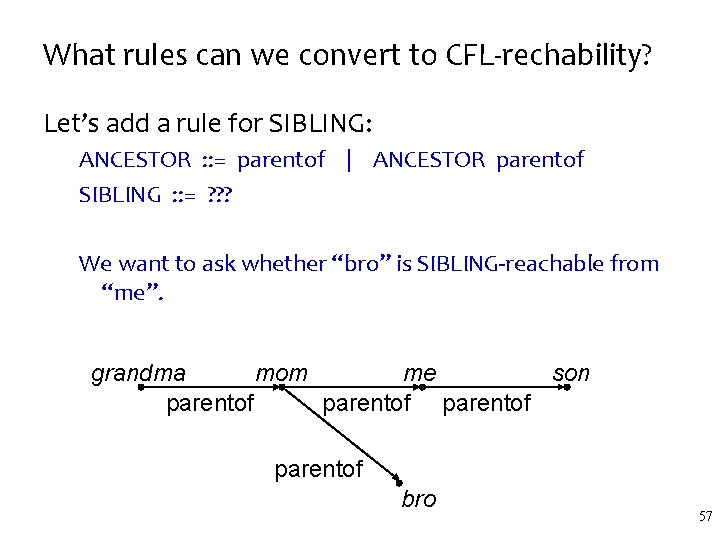What rules can we convert to CFL-rechability? Let’s add a rule for SIBLING: ANCESTOR : : = parentof | ANCESTOR parentof SIBLING : : = ? ? ? We want to ask whether “bro” is SIBLING-reachable from “me”. grandma mom me son parentof bro 57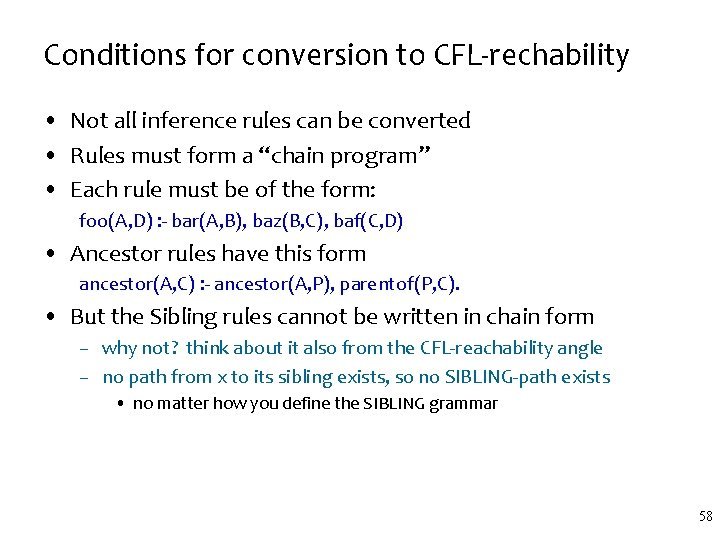Conditions for conversion to CFL-rechability • Not all inference rules can be converted • Rules must form a “chain program” • Each rule must be of the form: foo(A, D) : - bar(A, B), baz(B, C), baf(C, D) • Ancestor rules have this form ancestor(A, C) : - ancestor(A, P), parentof(P, C). • But the Sibling rules cannot be written in chain form – why not? think about it also from the CFL-reachability angle – no path from x to its sibling exists, so no SIBLING-path exists • no matter how you define the SIBLING grammar 58Andersen’s Algorithm with Chain Program converts the analysis into a graph parsing problem 59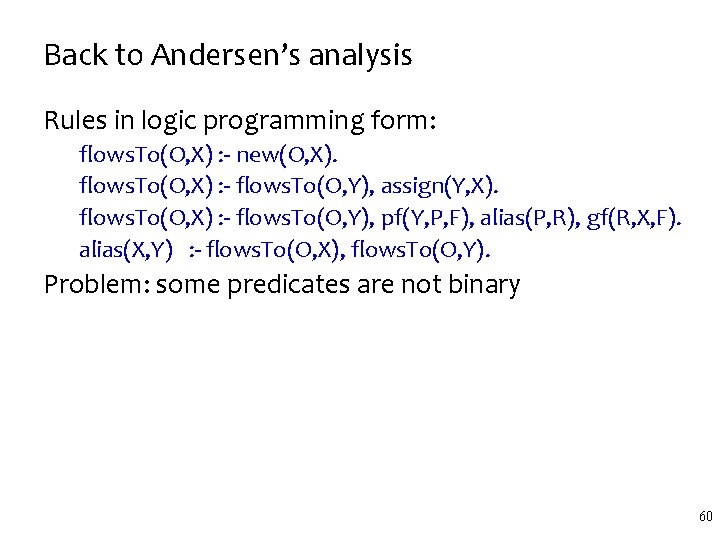Back to Andersen’s analysis Rules in logic programming form: flows. To(O, X) : - new(O, X). flows. To(O, X) : - flows. To(O, Y), assign(Y, X). flows. To(O, X) : - flows. To(O, Y), pf(Y, P, F), alias(P, R), gf(R, X, F). alias(X, Y) : - flows. To(O, X), flows. To(O, Y). Problem: some predicates are not binary 60Andersen’s algorithm inference rules Translate to binary form put field name into predicate name, must replicate third rule for each field in the program flows. To(O, X) : - new(O, X). flows. To(O, X) : - flows. To(O, Y), assign(Y, X). flows. To(O, X) : - flows. To(O, Y), pf[F](Y, P), alias(P, R), gf[F](R, X). alias(X, Y) : - flows. To(O, X), flows. To(O, Y). 61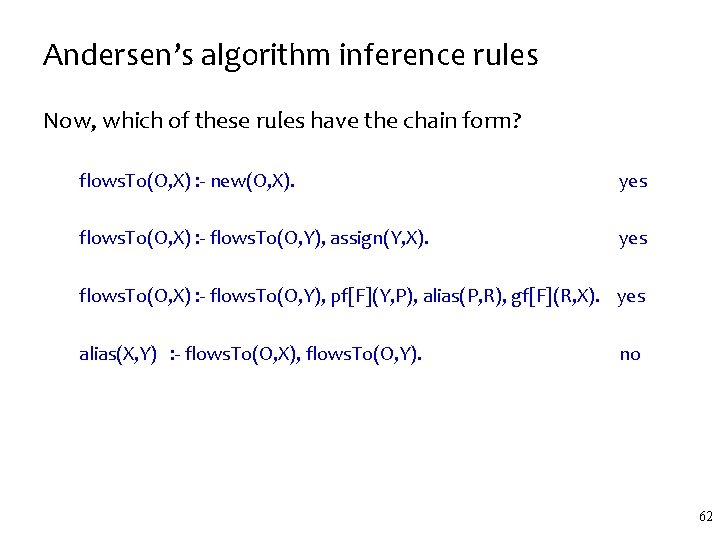Andersen’s algorithm inference rules Now, which of these rules have the chain form? flows. To(O, X) : - new(O, X). yes flows. To(O, X) : - flows. To(O, Y), assign(Y, X). yes flows. To(O, X) : - flows. To(O, Y), pf[F](Y, P), alias(P, R), gf[F](R, X). yes alias(X, Y) : - flows. To(O, X), flows. To(O, Y). no 62Making alias a chain rule We can easily make alias a chain rule with points. To. Recall: flows. To(O, X) : - points. To(X, O) : - flows. To(O, X) Hence alias(X, Y) : - points. To(X, O), flows. To(O, Y). If we could derive chain rules for points. To, we would be done. Let’s do that. 63Idea: add terminal edges also in opposite direction For each edge o new x, add edge x new-1 o – same for other terminal edges Rules for points. To will refer to the inverted edges – but otherwise these rules are analogous to flows. To What it means for CFL reachability? there exists a path from o to x labeled with s L(flows. To) there exists a path from x to o labeled with s’ L(points. To). 64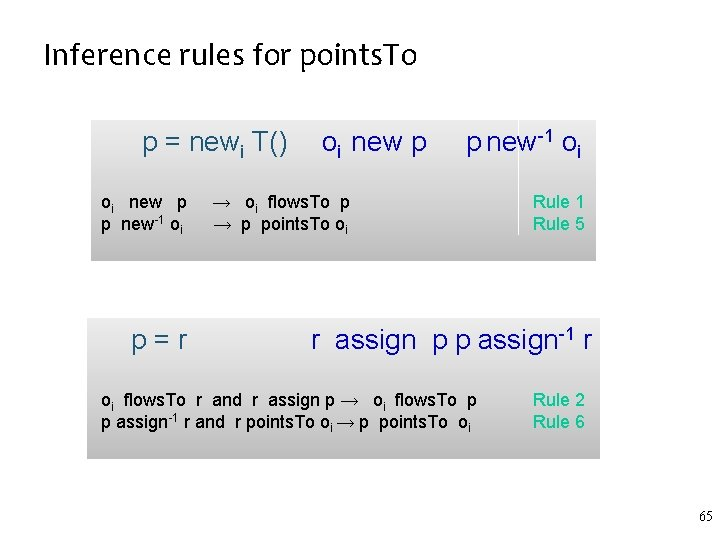Inference rules for points. To p = newi T() oi new p p new-1 oi p=r oi new p p new-1 oi → oi flows. To p → p points. To oi Rule 1 Rule 5 r assign p p assign-1 r oi flows. To r and r assign p → oi flows. To p p assign-1 r and r points. To oi → p points. To oi Rule 2 Rule 6 65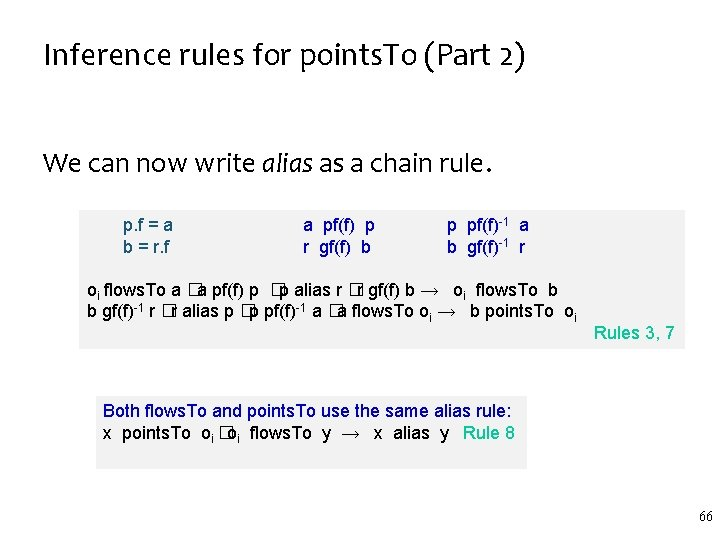Inference rules for points. To (Part 2) We can now write alias as a chain rule. p. f = a b = r. f a pf(f) p r gf(f) b p pf(f)-1 a b gf(f)-1 r oi flows. To a �a pf(f) p �p alias r �r gf(f) b → oi flows. To b b gf(f)-1 r �r alias p �p pf(f)-1 a �a flows. To oi → b points. To oi Rules 3, 7 Both flows. To and points. To use the same alias rule: x points. To oi �oi flows. To y → x alias y Rule 8 66The reachability language All rules are chain rules now – directly yield a CFG for flows. To, points. To via CFLreachability : flows. To points. To alias → → → → new flows. To assign flows. To pf[f] alias gf[f] new-1 assign-1 points. To gf[f]-1 alias pf[f]-1 points. To flows. To 67Example: computing points. To-, flows. Toreachability Inverse terminal edges not shown, for clarity. new n ssig a w x o 1 as si gn y gf[f] v pf[f] z new o 2 68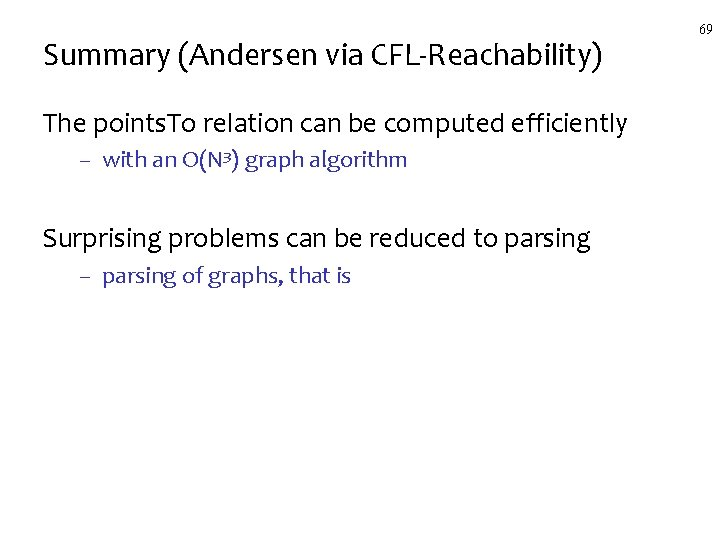Summary (Andersen via CFL-Reachability) The points. To relation can be computed efficiently – with an O(N 3) graph algorithm Surprising problems can be reduced to parsing – parsing of graphs, that is 69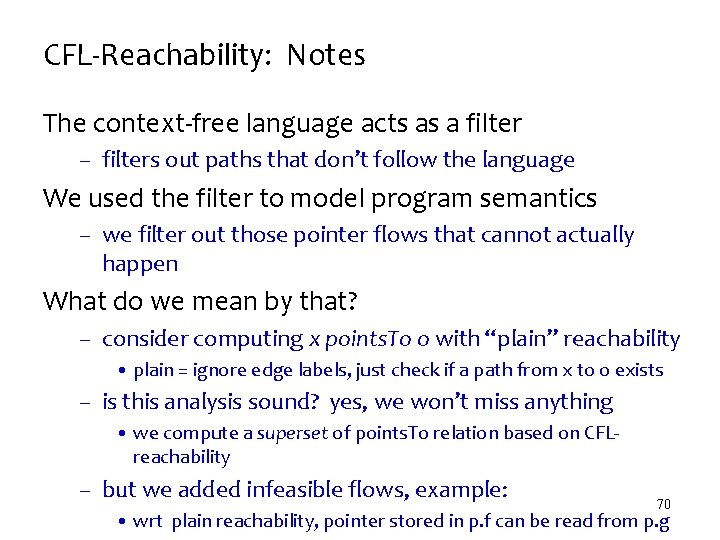CFL-Reachability: Notes The context-free language acts as a filter – filters out paths that don’t follow the language We used the filter to model program semantics – we filter out those pointer flows that cannot actually happen What do we mean by that? – consider computing x points. To o with “plain” reachability • plain = ignore edge labels, just check if a path from x to o exists – is this analysis sound? yes, we won’t miss anything • we compute a superset of points. To relation based on CFLreachability – but we added infeasible flows, example: 70 • wrt plain reachability, pointer stored in p. f can be read from p. g